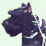# Calculation not working after using both pulldata() and user entered data - is it my syntax or something else?

213
1
05-19-2020 06:41 AMNew Contributor

I have a simple survey where my calculations are not working. It is either my syntax for the calculation or where it calculations are in the survey.

The Survey calculates Gallons Per Minute. The user selects a station which uses pulldata() to draw depth and width values from a separate table. Then they enter in two other values for a beginning and ending test dimension. Once the user enters in the two other values, a calculation needs to take place using the pulldata() values and the user entered values.

The equation I am trying to use is the following one out of an Excel spreadsheet.

IF(\$B\$6>0,\$B\$5*\$B\$6*(C11-C12)/12*7.48,3.14*(\$B\$5/2)^2*(C11-C12)/12*7.48))

B5 and B6 are pulldata() values in the Survey, C11 and C12 are the user entered values for start/finish dimensions in the Survey.

I know that excel formulas don't translate over to Survey123 completely so I am wondering if my syntax for the equation could be wrong in Survey, or it is how I have it set up that is preventing it from running the calculations.

I broke up the excel IF statement into two calculations

1. \$B\$5*\$B\$6*(C11-C12)/12*7.48

2. 3.14*(\$B\$5/2)^2*(C11-C12)/12*7.48

My versions of the excel formula in Survey

1. int(((\${well_length}*\${well_width})*(\${pump1_draw_startlevel}-\${pump1_draw_finishlevel})div12)*7.48)

2. int((3.14*(pow((\${well_length}div2),2))*(\${pump1_draw_startlevel}-\${pump1_draw_finishlevel})div12)*7.48)

Any help would be appreciated.

Tags (2)by